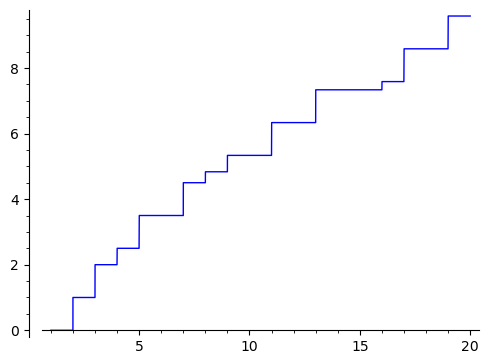# cannot convert log(x)/log(2) to int

If I define J(x) function as:

def J(x):
sum = 0
print(int(log(x)/log(2)))
for i in range(1, int(log(x)/log(2)) + 1):
sum = sum + 1 / i * prime_pi(x**(1/i))
return(N(sum))


when I try to plot it using:

p = plot(J(x), (x, 1, 20))
show(p)


I get the message:

cannot convert log(x)/log(2) to int


but if try J function acting on any float number I get a perfect value like:

sage: J(123.456789)
34.0333333333333


I have tried to replace int by floor but with the same result. Any help to solve the plotting problem will be welcome. Thank you.

edit retag close merge delete

Sort by » oldest newest most voted

Many such plotting problems are solved by using a callable function or a lambda function instead of a symbolic expression.

The problem is that J(x) tries to evaluate J with the symbolic variable x as an argument, and it cannot make log(x)/log(2) an integer while x stays symbolic.

To work around that, plot using J rather than J(x):

p = plot(J, (1, 20))
p


or use a lambda function:

p = plot(lambda x: J(x), (1, 20))
pThe function J could be rewritten as:

def J(x):
return sum(1/i * prime_pi(x**(1/i))
for i in range(1, int(log(x)/log(2)) + 1))

more